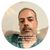QlikView App Dev

Discussion Board for collaboration related to QlikView App Development.

Announcements
QLIKWORLD LIVE! MAY 16 - 19TH, EARLY BIRD DISCOUNTS! REGISTER TODAY
cancel
Showing results for
Did you mean:Contributor II

Duplicates counting and flagging

Dear all,

I got stuck on what seemed a simple problem.

I have a table A with fields

A.id, ... ,

A.text

Lets say that it looks like this:

A.idA.text
1ALPHA
2BRAVO
3ALPHA
4CHARLIE

In a pivot chart, I would like to show, which texts have duplicates and count the number of duplicates:

A.textFlag multiple

ALPHA

1
BRAVO0
CHARLIE0
SUM1

I tried if-clauses:

if( count ({<...>}A.text)  - count (distinct {<..>}A.text)=0, 0,1)

Nice and simple. That does in fact deliver the correct result - but it fails to work in summing up. As QV considers the if-clause for all the datasets above, I will always get a "1" instead of the number of terms with duplicates ("Doubletten").

So, I also tried aggr with an aggregation level ("Team number") several tables away

sum(aggrif( count ({<...>}A.text)  - count (distinct {<..>}A.text)=0, 0,1) ), A.text)

And here it starts getting messy, as some values are correct, some are not and the whole thing changes with the selections I make. Weird. Unfortunatly, I can not post the data here...

I thought of mapping the data into a separate table by script, but there must be a somewhat more elegant way to this.

Have I overlooked a much more simple way?

How would you approach such a task?

Any help greatly appreciated,

best regards,

Boris

1 Solution

Accepted SolutionsCreator III

Try this,

Pivot chart with,

Dimension: A.Text

Expression: =sum(aggr(if(Count(A.Text)<=1,0,1),A.Text))

From Presentation tab 'UnCheck' the 'Suppress Zero-Values' option

I am getting below result9 RepliesCreator III

Try this,

Pivot chart with,

Dimension: A.Text

Expression: =sum(aggr(if(Count(A.Text)<=1,0,1),A.Text))

From Presentation tab 'UnCheck' the 'Suppress Zero-Values' option

I am getting below resultMVP

In a pivot chart, I would like to show, which texts have duplicates and count the number of duplicates

What happens if you simply select A.Text as dimension and Count(A.id) as the expression?Creator III

This will show the duplicate count of A.TextMVP

I mean if the exact expression we use like if(Count(A.id)>1,1,0) to denote duplicate as 1, shouldn't this simple expression work or not?Creator III

it works, but sub total is coming to 1. Not the total of duplicates.MVP

Got it, to get the right total we need to aggregate the results in expression. ThanksMVP

what could be the output for this data with correct total?

 ID TEXT 1 A 2 B 3 A 4 A 5 B 6 CContributor II
Author
 TEXT Flag A 1 B 1 C 0 total 2

The idea is to figure out, how many texts (which correspond to companies in my QV-data) overlap in various fields of business.

I found the solution: sum(aggr(if(Count(A.Text)<=1,0,1),A.Text)) as suggested by Mayuresh above works fine. My question was not sufficiently precise, I am a bit of an aggr-rookie... 🙂

But as I needed an aggregation in a pivot chart, I had to use a second aggr level, else the results would vary according to the filters applied.

So, I used count(aggr(sum(aggr(count (distinct A.text), A.id)),Team_Number))

This may not be the most elegant solution, but it works.Contributor II
Author

Thank you, Mayuresh!

Your solution works within the table, but I actually applied a different version.

Your suggestion got me there by getting me off the idea of substracting count to count distinct.

Instead, I now use count (aggr (sum(aggr (count (distinct A.text), A.id)), Team_Number))

I am a bit of an aggr-newbie, so I got stuck on using just one aggr-level in a pivot table and then got confused about the verying results all according to the filters applied. .best regards,

BorisCommunity Browser